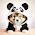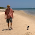Wednesday, May 30, 2018

Broken symmetry, order, and entropy

One of the greatest joys of teaching is having students ask questions that you do not know the answer to. In the last week of the course PHYS2020 Thermodynamics and Condensed Matter Physics for second year undergrads at UQ, I give two lectures about critical points, universality, critical exponents, broken symmetry, order parameters, and Landau theory.

Many students find this quite challenging. However, I think it is important that students be exposed to two of the most important ideas of theoretical physics from the twentieth century: broken symmetry and universality. Furthermore, there is no technical reason why second year undergrads cannot learn this material. Since the text, Thermal Physics by Schroeder, does not cover this material we have finally settled on a chapter from a book by Hoch.

After my last lecture, a student asked an excellent question along the lines of
"Why is it that broken symmetry occurs at lower temperatures?
How is this related to entropy and order?"

This led me to wondering whether there were any rigorous results that answer the question. I could not find anything in a quick search.
Do you know of anything?

I was wondering whether something like the following conjecture was true:
Conjecture. Consider a physically reasonable Hamiltonian H for an infinite system. Suppose H is invariant under some symmetry group G. Let rho(T) be the equilibrium density matrix at temperature T. Then for sufficiently large T, rho(T) is also invariant under G.
Maybe this is equivalent to
Lemma. At sufficiently high temperatures, the von Neumann entropy S (rho) = - Tr( rho ln (rho)) is maximal if rho is invariant under G.
This looks to me like the kind of thing that people like Elliot Lieb, David Ruelle, Y. Sinai, ... might have tackled at some point.

I welcome ideas and suggestions.

1.Even at low temperature the system is invariant under G, in the absence of the (infinitesimal) field selecting how G is broken. In particular, in the low T phase, the Gibbs free energy (which depends on the magnetization M) takes the same value for all magnetization -m0^+). This is because the free energy is NOT a double well in the thermodynamic limit because it has to be convex.
(Magnetization |M|<m are allowed because the cost of having domains is subvolumic and thus does not count in the thermodynamic limit. All these complication arises because there is a first order transition (which involves mixed states) as h goes to 0^- to 0^+.)
I don't think discussing the entropy is too relevant, since we are talking about systems in contact with a thermal bath.

2.There's a formal subtlety that needs to be properly dealt with. Clearly for all finite systems where the Hamiltonian is bounded, the density matrix at any temperature commutes with the Hamtonian. One must first choose a formalism that deals with the limit correctly.

3.There are subtle examples from soft matter, such as the existence of lower consolute temperatures, where phase separation happens as the temperature increases. These are usually due to fairly subtle entropic effects.

1.Gatuam,

Thanks for the comment.

I agree that there are examples where as the temperature increases a phase transition takes place to a state of lower entropy.

However, my conjecture would claim that if one goes to a sufficiently high temperature, then even in such systems, the system will enter a phase with no broken symmetry.

4.Hi Ross, let me comment here since I found myself stumped by a related question recently as well. I appreciate the model of discussion you set by your blog.

It makes sense that symmetry breaking should typically occur at lower temperature. Of course at low T the entropy can't contribute as much to lowering the free energy F=E-TS. When symmetry is preserved the system samples all the different states related by the symmetry (e.g. a liquid enjoys the entropy of all the different spatial configurations). When symmetry is broken the system is restricted to just the one spontaneously chosen configuration and there is no entropy gained in that degree of freedom (a solid is frozen).

The weird thing is that this is not a theorem, and indeed there are counterexamples. A famous one is in the phase diagram of He3, where the solid can *melt* as you lower the temperature. The explanation, which goes under the name "Pomeranchuk cooling", shows that the entropy is indeed still lowered even as the solid melts: this is due to the interplay of atomic positional ordering with the spin configurations. The total entropy of the solid can here be larger than that of the liquid.

So finite temperature symmetry breaking is still set by the entropy, but our intuition about symmetry-breaking reducing entropy might not hold if multiple degrees of freedom have a complicated dependency.

1.Itamar, thanks for the helpful comment.

I agree that there are temperature ranges where lowering the temperature can reduce the symmetry breaking and can increase the entropy. 3He is a nice example.

However, my question is where at sufficiently high temperatures it is always true that there is no broken symmetry and the entropy is maximal for that state.

5.In the book by Anderson, Principles of Condensed Matter Physics, there is very lengthy and physical discussion of this issue. It should be noted that symmetry breaking is not exclusive to the quantum world.